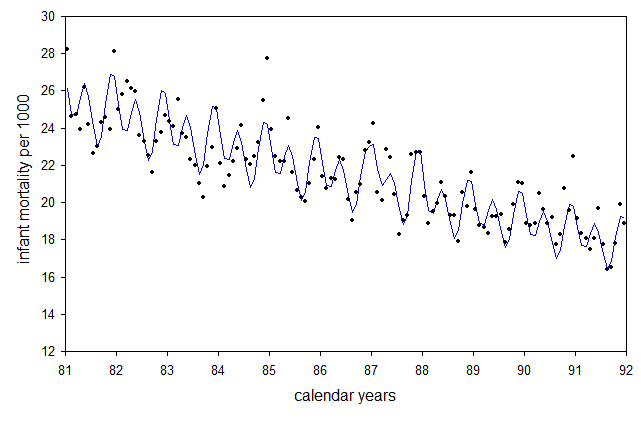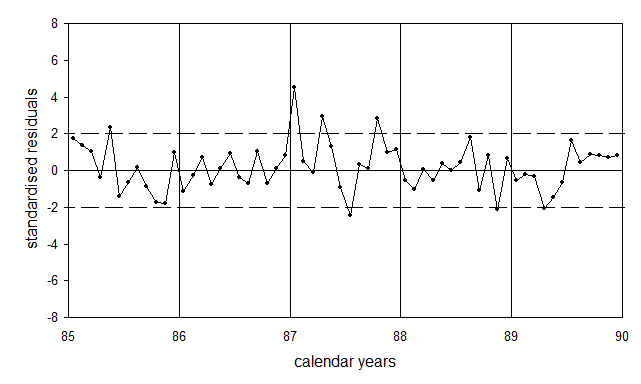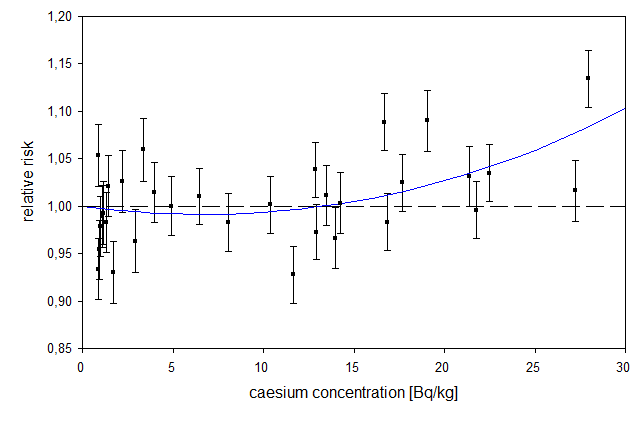Infant mortality in Poland

Poland which has borders with Belarus and Ukraine is likely to be more contaminated from the Chernobyl fallout than Germany. In addition, Poland has a rather large population of nearly 40 mio. Data from Poland may support the findings of increased perinatal mortality data in Germany.

Unfortunately I could not get monthly data of perinatal mortality from Poland. Therefore monthly data of infant mortality, 1981 through 1991, were used.

Data evaluation

For the data analysis, a non-linear regression model is applied (statistical package R, function  nls):

fm <- nls(p~1000/(1+1/exp(c0+c1*x+c2*cos(2*pi*(x-c3))+c4*cos(2*pi*(2*x-c5))+c6*cs+c7*cs^2)),

where x is time where x=t-1980 and t is calendar year.
To allow for seasonal changes, two periodic terms with periods of 6 and 12 months are introduced (parameters c2-c5). cs(x) are monthly averages of the caesium burden of women during pregnancy from consumption of cow milk, delayed by seven months.

Regression results

The model fit to the data shows large overdispersion (SSE=332.2; df=124, dispersion parameter OD=2.68). Therefore a regression for a restricted time interval, 1985-1989, was conducted. It yielded SSE=80.2; DF=52; OD=1.54.
The following table contains the parameter estimates and the respective p values.

 parameter estimate SE t-value p-value c0 -3.6035 0.0268 -134.3 0.0000 c1 -0.0342 0.0037 -9.21 0.0000 c2 0.0332 0.0076 4.378 0.0001 c3 0.0344 0.0345 0.997 0.3233 c4 0.0639 0.0071 9.003 0.0000 c5 0.8007 0.0176 45.56 0.0000 c6 -0.2608 0.2254 -1.157 0.2527 c7 1.9611 1.0112 1.939 0.0579

The linear caesium term has a negative sign, the quadratic term a positive sign.
The combined effect of the linear and the quadratic caesium terms is not significant (p=0.014; F-test).
A regression with only a linear caesium term yields p=0.0245.

Thus infant mortality in Poland - like perinatal mortality in Germany - is associated with the delayed caesium burden of pregnant women.Fig.1: Monthly data of infant mortality in Poland, 1981-1991, and secular trend (blue line).

Residuals

Figure 2 shows the residuals, ie the deviations of the data from the model fit, in units of standard deviations (standardised residuals). In January 1987 and in April 1987 infant mortality rates are significantly increased.Fig.2: Residuals of infant mortality rates in Poland, 1985-1989. The broken lines indicate the range of two standard deviations.

Dose-response relationship

Figure 3 displays the association between infant mortality and delayed caesium burden. The y-axis shows the ratio of observed to expected infant mortality rates (relative risk).Fig.3: Ratio of observed to expected infant mortality rates (relative risk) as a function of the delayed caesium burden of pregnant women. The blue line is the result of a regression with a linear-quadratic model. The error bars are standard deviations.